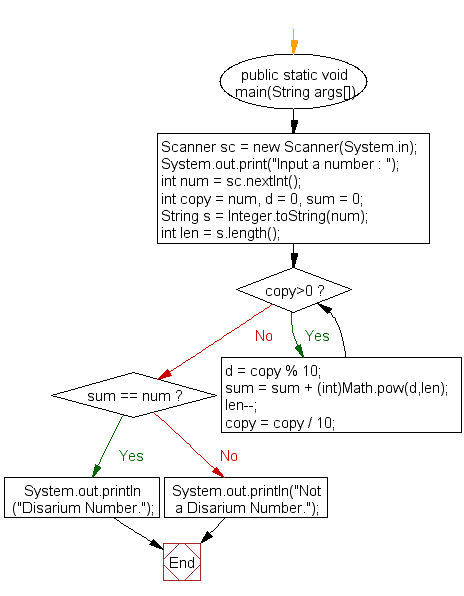﻿ Java exercises: Check whether a given number is a Disarium number or unhappy number - w3resource# Java Exercises: Check whether a given number is a Disarium number or unhappy number

## Java Numbers: Exercise-11 with Solution

Write a Java program to check whether a given number is a Disarium number or unhappy number.

A Disarium number is a number defined by the following process:
Sum of its digits powered with their respective position is equal to the original number.
For example 175 is a Disarium number:
As 11+32+53 = 135
Some other DISARIUM are 89, 175, 518 etc.
A number will be called Disarium if the sum of its digits powered with their respective position is equal with the number itself. Sample Input: 135.

Test Data
Input a number : 25

Pictorial Presentation:Sample Solution:

Java Code:

``````import java.util.Scanner;

public class Example11 {

public static void main(String args[])
{
Scanner sc = new Scanner(System.in);
System.out.print("Input a number : ");
int num = sc.nextInt();
int copy = num, d = 0, sum = 0;
String s = Integer.toString(num);
int len = s.length();

while(copy>0)
{
d = copy % 10;
sum = sum + (int)Math.pow(d,len);
len--;
copy = copy / 10;
}

if(sum == num)
System.out.println("Disarium Number.");
else
System.out.println("Not a Disarium Number.");
}
}
```
```

Sample Output:

```Input a number : 25
Not a Disarium Number.
```

Flowchart:Java Code Editor:

What is the difficulty level of this exercise?

﻿

## Java: Tips of the Day

Array vs ArrayLists:

The main difference between these two is that an Array is of fixed size so once you have created an Array you cannot change it but the ArrayList is not of fixed size. You can create instances of ArrayLists without specifying its size. So if you create such instances of an ArrayList without specifying its size Java will create an instance of an ArrayList of default size.

Once an ArrayList is full it re-sizes itself. In fact, an ArrayList is internally supported by an array. So when an ArrayList is resized it will slow down its performance a bit as the contents of the old Array must be copied to a new Array.

At the same time, it's compulsory to specify the size of an Array directly or indirectly while creating it. And also Arrays can store both primitives and objects while ArrayLists only can store objects.

Ref: https://bit.ly/3o8L2KH